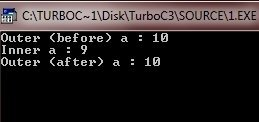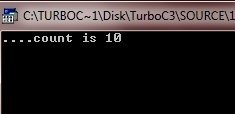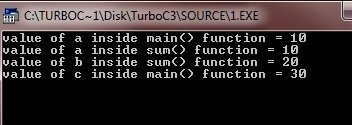# C Scope Rules

A scope in C is a region of the program where the defined variable can have its existence and beyond that, variable can't be accessed. There are the following three places to declare variables in C:

1. Inside the function or in a block which is also called as local variables
2. In the definition of function parameters which is also called as formal parameters.
3. Outside of all the functions which is also called as global variables.

In C programming, Scope of an identifier is the part of the program where the identifier may directly be accessible. C scope rules tells rule of the scope of a variable that what is the scope of that variable. For example, local scope, global scope or just a formal parameters.

If the scope of any variable is local, then it can only be accessible locally. For example, if a variable is declared inside a function, then the scope of that variable is only in that function. It means, outside that function, there is no any variable of that name until a new variable is defined with the same name. And if the scope of any variable is global, then the variable can be accessible from the whole program or can universally be accessible. And in case of formal parameters, it is just like a local variable that can be only known to that function.

So if you want to use the same variable throughout the whole program from one function to other and from one place to other, then use the global variable, means define the variable globally. And if you want to use a variable locally, it means you want to use the variable only for some local work and then want to destroy that variable, then use the local variable. Let's explain C scope rules in detail one by one from local variable to formal parameters.

Read Carefully the below topic to understand scope rules in C.

## Local Variables

Local Variables are the variables declared inside a block or a function. Since the variable declared and used locally, so that it is called as local variables. Local variables can only be used inside a block or a function where the variables are declared. Outside that function or block it becomes unknown and it will be destroyed after exiting that function or block.

So if you want to use a variable only for limited time or only for some specific purpose or only for some place or in some function or in some block, then simply use the local variable. If you enter that function or block where the variable is declared, then that variable works and destroyed automatically after going out from that function or block. Let's look at the following C program carefully :

```/* C Scope Rules - Local Variable Example */

#include<stdio.h>
#include<conio.h>
void main()
{
int a;
clrscr();

a = 10;
printf("Outer (before) a : %d\n", a);
if(a==10)
{
int a;   // this a hides the outer a
a = 9;
printf("Inner a : %d\n", a);
}
printf("Outer (after) a : %d\n", a);

getch();
}```

When the above C program is compile and executed, it will produce the following output:Here, first value of a is 10, which is outside the if block and on going into if block (local), we initialized the value of a as 9 after declaring it. So when we exit the if block, the value of a will be destroyed and holds the outer value i.e., 10 as shown in the output figure. Always keep in mind about this change.

Local variables can be used by the local statements only where they are declared i.e. the scope of that variable is local, means the variable can't be accessible from outside the blocks where they are declared. Variables becomes unknown outside the block since the scope of the variable is local only this is because a local variable only created upon entry to that block and destroyed automatically on exits. Whereas the scope of the global variable is throughout the program since it is created when the program starts execution and destroyed at the time when program execution stops.

## Global Variables

Global variables are the variables which are defined outside of a function, usually on top of the program ie. above the main() function. Global variable known throughout the program. Global variables holds their values throughout the program. So the Global variables are accessible throughout the program from outside the functions or blocks to inside the functions or blocks. In short, you can access global variable from anywhere in the program.

Important - In case if the name of the global variable and local variable will be same, then the priority will first goes to the local variable. It means first local variable will be used.

A global variable can be accessed by any function or blocks. Go through the following C program that tells you all about the global variables scope. Read the following C program carefully and watch the output :

```/* C Scope Rules - Global Variable Example */

#include<stdio.h>
#include<conio.h>

int count;     // this count variable is global

void fun1(void);
void fun2(void);

void main()
{
clrscr();

count = 10;
fun1();

getch();
}

void fun1(void)
{
fun2();
printf("count is %d", count);   // this will print 10
}

void fun2(void)
{
int count;   // this is local variable having first priority
for(count=1; count<5; count++)
{
putchar('.');
}
}```

When the above c program is compile and executed, it will produce the following output:The scope of the global variables is throughout the program. And if you declare and initialize the value to the variable with same name then local variable is given as high priority. So, the value given to the variable inside the local block or functions will be holds that value until that block or functions closed. It means local variables will be created that hides the global variable and it will be create upon the entry in the block and destroyed at exit from the block or functions.

So a program can have same name for local and global variables but the value of a local variable inside a function will take preference.

## Formal Parameters

Function parameters or formal parameters are treated, same as local variables within that function and they will take preference over the global variables. Following is an example:

```/* C Scope Rules - Format Paramter Example */

#include<stdio.h>
#include<conio.h>

int a = 20;    // global variable declaration

void main()
{
/* local variable declaration in main() function */
int a = 10;
int b = 20;
int c = 0;
clrscr();

printf("value of a inside main() function = %d\n", a);
c = sum( a, b);
printf("value of c inside main() function = %d\n", c);

getch();
}

int sum(int a, int b)
{
printf ("value of a inside sum() function = %d\n", a);
printf ("value of b inside sum() function = %d\n", b);

return a + b;
}```

On compile and executing the above code, it will produce the following result:Here, variable a and b inside the function sum() in the main() function is just a formal parameters and a holds 10, b holds 20. So the value of a and b is passed in the sum() function. The value of a and b is printed inside the sum function. Then the addition of a and b is return by the sum() function which is initialized to c. Then c is printed on the screen which is 30

## Initializing Local and Global Variables

When a local variable is defined, it is not initialized by the system, you must have to initialize it yourself. Global variables are initialized automatically by the system when you define them as follows:

Data Type Initial Default Value
char '\0'
int 0
float 0
double 0
pointer NULL

Now you learned about C Scope Rules. In next tutorial, you will learn about Arrays in C programming.

Tools
Calculator